##Hyperbola from Rectangular

The simplest hyperbola (standard) is the one defined through the graph of the function y = 1/x (gray). All other hyperbolas are congruent to the hyperbolas resulting from the previous one through an affinity given by the transformation [x'=ax, y' =cx+by]. The matrix of this linear transformation has determinant ab, hence the asymptotic triangles of the resulting hyperbola have area 2ab (see HyperbolaRectangular.html ).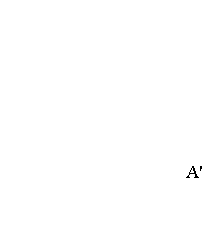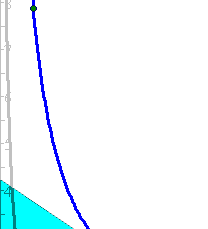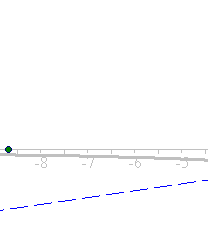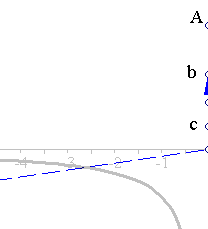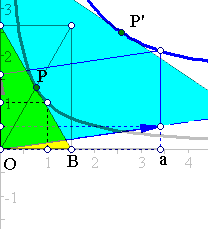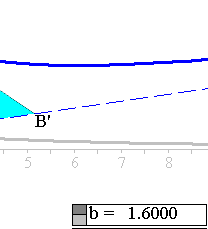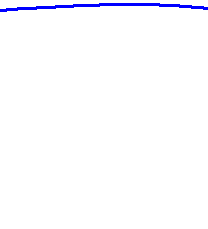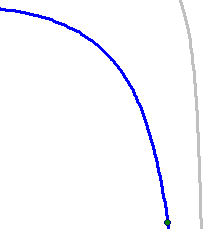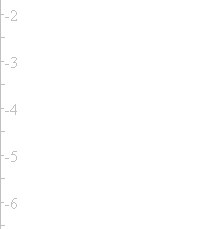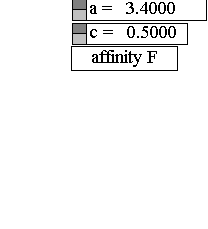The picture shows the standard hyperbola (gray) and its image (blue) through the transformation F: [x'=ax, y' =cx+by]. P' is the image of P. The asymptotic triangle OAB of the standard hyperbola is mapped to the asymptotic triangle OA'B' of the image-hyperbola. The area of OAB is 2 and that of OA'B' is 2 multiplied by the determinant of the matrix defining the transformation. This being ab, the area of the asymptotic triangle OA'B' is 2ab. The points of the hyperbola can be represented as the graph of the function y' = (c/a)x' + (ab)/x'.